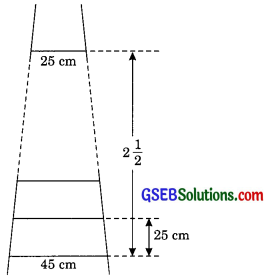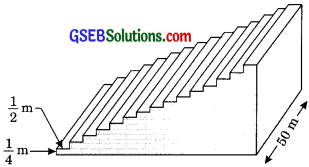# GSEB Solutions Class 10 Maths Chapter 5 Arithmetic Progressions Ex 5.4

Gujarat Board GSEB Solutions Class 10 Maths Chapter 5 Arithmetic Progressions Ex 5.4 Textbook Questions and Answers.

## Gujarat Board Textbook Solutions Class 10 Maths Chapter 5 Arithmetic Progressions Ex 5.4

Question 1.
Which term of AP 121, 117, 113, …………..is its first negative term?
[Hint: Find n for an < 0 ]
Solution:
The given AP is 121, 117, 113, ……………
Here a = 121,
d = 117 – 121 = – 4
Now we let nth term of the given AP be the negative term then
an < 0
a + (n – 1) d < 0
121 + (n – 1)( – 4) < 0
121 < (n – 1) x 4
n – 1 > $$\frac { 121 }{ 4 }$$
n > $$\frac { 125 }{ 4 }$$ + 1
= n > 31 $$\frac { 1 }{ 4 }$$
Least Integral value of n is 32.
Hence 32nd term is first negative term of given AP.Question 2.
The sum of the third and the seventh terms of an AP is 6 and their product is 8. Find the sum of first. sixteen terms of the AP, respectively.
Solution:
Let a and d be the first term and common difference of the given AP, respectively.
According to the problem,
Third term + seventh term = 6
a + 2d + a + 6d = 6
2a + 8d = 6
a + 4d = 3 …………… (1)
(dividing by 2)
and third term x seventh term = 8
(a + 2d)(a + 6d) = 8
From eqn. (1) we get
a = 3 – 44
Putting value of a in eqn. (2)
= (3 – 4d + 2d)(3 – 4d + 6d) = 8
(3 – 2d)(3 + 2d) = 8
9 – 4d2 = 8
– 4d2 = -1
d2 = $$\frac { 1 }{ 4 }$$
d = ± $$\frac { 1 }{ 2 }$$
Casel: When d = $$\frac { 1 }{ 2 }$$
Putting the value of d in eqn. (1)
a + 4 x $$\frac { 1 }{ 2 }$$ = 3
a + 2 = 3
a = 1
For, n = 16
Sum of first sixteen terms of AP = S16
Sn = $$\frac { n }{ 2 }$$ [2 x a + (n – 1)d]
S16 = $$\frac { 16 }{ 2 }$$ [2 x 1+ (16 – 1) ]
= 8 [2 + 15 x $$\frac { 1 }{ 2 }$$]
= 8 $$\left[ \frac { 19 }{ 2 } \right]$$ = 4 x 19 = 76
Case II: When d = $$\frac { – 1 }{ 2 }$$
Putting value of d = $$\frac { – 1 }{ 2 }$$ in eqn (1)
We get
a + 4d = 3
a + 4 x ($$\frac { – 1 }{ 2 }$$) = 3
a – 2 = 3
a = 5
For, n = 16
Sum of first sixteen terms of AP = S16
Sn = $$\frac { n }{ 2 }$$ [2a + (n – 1)d]
S16 = $$\frac { 16 }{ 2 }$$ [2 x 5 + (16 – 1) x ($$\frac { – 1 }{ 2 }$$)]
= 8[10 – $$\frac { 15 }{ 2 }$$] = 8$$\frac { 5 }{ 2 }$$
= 4 x 5 = 20Question 3.
A ladder has rungs 25 cm apart. (see Fig.). The rungs decrease uniformly in length from 45 cm at the bottom to 25 cm at the top. If the top and the bottom rungs are 2$$\frac { 1 }{ 2 }$$ m apart, what is the length of the wood required for the rungs?
[Hint: Number of rungs = $$\frac { 250 }{ 25 }$$ + 1]Solution:
Number of rungs = + 1
= 10 + 1
= 11
It is given that the length of rungs decreases
uniformly from 45 cm at bottom to 25 cm at the
top. So the length of rungs form an AP of first
term a = 45 cm and last term is 25 cm
Here a = 45 cm
d = 25cm
n = 11
Total length of wood required for rungs
= [a + 1] = – [45 + 25]
= $$\frac { 11 }{ 2 }$$ x 70 = 11 x 35
= 385 cm = 3.85 metre.Question 4.
The houses of a row are numbered consecutively from 1 to 49. Show that there is a value of x such that the sum of the numbers of the houses preceding the house numbered x is equal to the sum of the numbers of the houses following it.
Find this value of x.
[Hint: Sx-1 = S49 – Sx]
Solution:
The consecutive numbers on the houses of a row are 1, 2, 3 49. This list of numbers form an AP.
Here a = 1,
d = 2 – 1 = 1
Sn = $$\frac { n }{ 2 }$$ [2a + (n – 1)d]
Sx – 1 = $$\frac { x-1 }{ 2 }$$ [2 x 1 + (x – 1 – 1) x 1]
Sx – 1 = ($$\frac { x-1 }{ 2 }$$) [2 x 1 + (x – 1 – 1) x 1]
= $$\frac { \left( x\quad -1 \right) }{ 2 }$$ [2 + x – 2]
Sx – 1 = $$\frac { \left( x\quad -1 \right) }{ 2 }$$ x x
S49 = $$\frac { 49 }{ 2 }$$ [2 x 1 + (49 – 1) x 1]
=$$\frac { 49 }{ 2 }$$ [2 + 48]
= $$\frac { 49 }{ 2 }$$ x 50
S49 = 49 x 25
Sx = $$\frac { x }{ 2 }$$ [2 x 1 + (x -1) x 1]
$$\frac { x }{ 2 }$$ [2 + x – 1]
Sx = $$\frac { x }{ 2 }$$ [x + 1]
According to the problem,
Sx-1 = S49 – Sx
$$\left( \frac { x-1 }{ 2 } \right) x$$x = 49 x 25 – $$\frac { x }{ 2 }$$ x (x + 1)
$$\frac { \left( x-1 \right) x }{ 2 }$$ + $$\frac { x }{ 2 }$$(x+1) = 49 x 25
$$\frac { x }{ 2 }$$[x – 1 + x + 1] = 49 x 25
$$\frac { x }{ 2 }$$ x 2x = 49 x 25
x2 = 49 x 25
x = 7 x 5
x = 35Question 5.
A small terrace at a football ground comprises of 15 steps each of which is 50 m long and built of solid concrete. Each step has a rise of $$\frac { 1 }{ 4 }$$ m and a tread of $$\frac { 1 }{ 2 }$$ m. (see figure). Calculate the total volume of concrete required to build the terrace.
[Hint: Volume of concrete required to build the first step = $$\frac { 1 }{ 2 }$$ x $$\frac { 1 }{ 2 }$$ x 50 m3]Solution:
We observe that the length and breadth of each step is 50 m and $$\frac { 1 }{ 2 }$$m respectively.
Height of first step = $$\frac { 1 }{ 2 }$$ m
Height of second step = $$\frac { 1 }{ 4 }$$ m +$$\frac { 1 }{ 4 }$$ m = $$\frac { 2 }{ 4 }$$ m
Height of third step = $$\frac { 3 }{ 4 }$$ m and so on.
Let us consider the volume of concrete steps are
V1, V2, V3, …………… V15
Hence V1 = l x b x h = $$\left( 50x\frac { 1 }{ 2 } x\frac { 1 }{ 4 } \right)$$m3
V2 = $$\left( 50x\frac { 1 }{ 2 } x\frac { 2 }{ 4 } \right)$$m3
V3 = $$\left( 50x\frac { 1 }{ 2 } x\frac { 3 }{ 4 } \right)$$m3
V4 = $$\left( 50x\frac { 1 }{ 2 } x1 \right)$$m3 and so on.
Total volume of concrete required
V = V1 + V2 + V3 + V4 + …………. + V15
= $$\left\{ 50x\frac { 1 }{ 2 } x\frac { 1 }{ 4 } \right\}$$ + $$\left\{ 50x\frac { 1 }{ 2 } x\frac { 2 }{ 4 } \right\}$$ + $$\left\{ 50x\frac { 1 }{ 2 } x\frac { 3 }{ 4 } \right\}$$ + ………… + $$\left\{ 50x\frac { 1 }{ 2 } x15x\frac { 15 }{ 4 } \right\}$$m
= 50 x $$\frac { 1 }{ 2 }$$ $$\left[ \frac { 1 }{ 4 } +\frac { 2 }{ 4 } +\frac { 3 }{ 4 } +………..+\frac { 15 }{ 4 } \right]$$ m3
= 50 x $$\frac { 1 }{ 2 }$$ x $$\frac { 1 }{ 4 }$$ [1 + 2 + 3 + ………….. + 15] m3
= $$\frac { 25 }{ 4 }$$ $$\left[ \frac { 15(15+1) }{ 2 } \right]$$
= $$\frac { 25 }{ 4 }$$ x $$\frac { 15×16 }{ 2 }$$
(sum of n terms of AP = $$\frac { n(n+1) }{ 2 }$$)
= 25 x 15 x 2
= 750 m3